Go back to  'Circles'

 1 Introduction to Circles 2 What is a Radius? 3 Formulas for Radius 4 Radius of a Circle Calculator 5 Equation of a Circle 6 Radius of a Curve 7 Important Notes on Radius 8 Solved Examples on Radius 9 Practice Questions on Radius 10 Challenging Questions on Radius 11 Maths Olympiad Sample Papers 12 Frequently Asked Questions (FAQs)

We at Cuemath believe that Math is a life skill. Our Math Experts focus on the “Why” behind the “What.” Students can explore from a huge range of interactive worksheets, visuals, simulations, practice tests, and more to understand a concept in depth.

Book a FREE trial class today! and experience Cuemath’s LIVE Online Class with your child.

## Introduction to Circles

A circle is a collection of points on a two-dimensional plane, which are equidistant from the centre point '$$O$$'.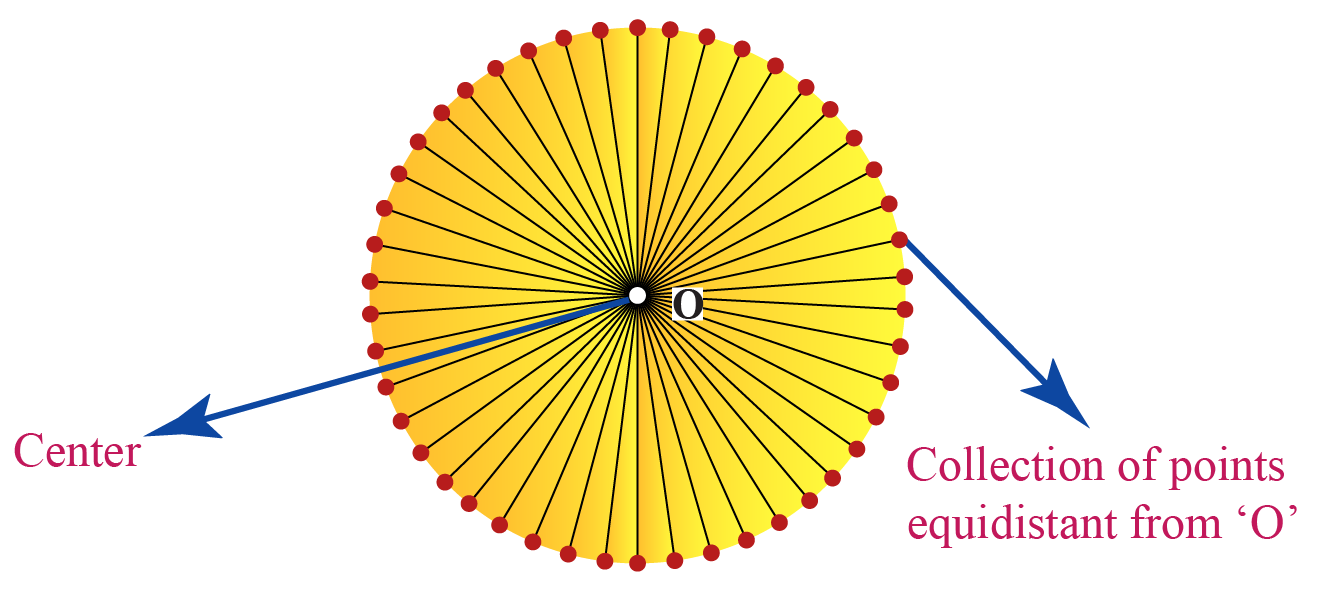The distance from the center point to any endpoint on the circle is the radius.

The size of the circle changes when the length of the radius varies.

You can observe this change by dragging the blue endpoint outwards on the circle.

The radius of a circle definition is the length of the line segment from the center of a circle to a point on the circumference of the circle.

A circle can have many radii (the plural form of radius) and they measure the same.

Radius of a circle is generally abbreviated as ‘$$r$$’.

Look at this image: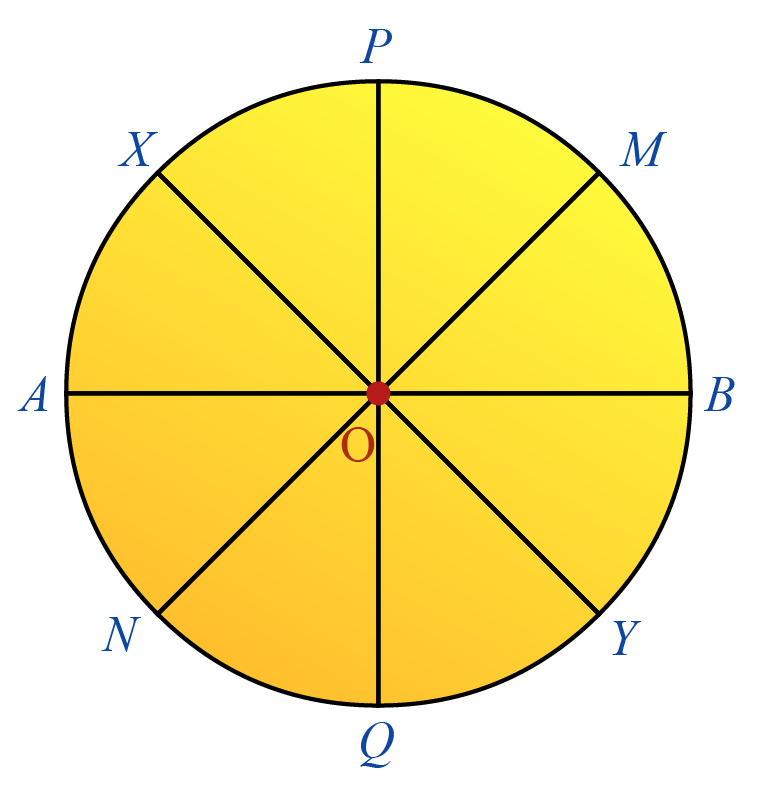The points $$A,\,B,\,M,\,N,\,P,\,Q,\,X,\,Y$$ lie on the boundary of the circle.

These points are equidistant from the center $$O$$.

$$OA=OB=OM=ON=OP=OQ=OX=OY$$

### Diameter of a Circle

Diameter is the longest chord of a circle.

The radius of a circle definition can be used to understand the diameter of a circle.

The diameter is twice the length of the radius.

 Diameter of a circle$$=2r$$

### Circumference of a Circle

The perimeter of the circle is called the circumference. It is the boundary of the circle.

Look at this simulation to understand the relationship between the radius and the circumference of a circle.

Drag the radius between $$1 \: \text{cm}$$ to $$5 \:\text{cm}$$.

The circumference of a circle in terms of its radius is expressed as:

 $$C = 2\pi r$$

Where;

• $$C$$ is the circumference
• $$r$$ is the radius
• $$\pi$$ is the constant $$3.14159...$$

### Area of a Circle

Area of a Circle is the area occupied by the circle.

The relationship between the radius and area is given by:

 Area of the circle $$= \pi r^2$$

Where;

• $$r$$ is the radius
• $$\pi$$ is the constant $$3.14159...$$

Radius can be calculated when the diameter, the area, or the circumference is known. Refer to the formulas below to calculate the radius.

The radius of a circle formula when the diameter is known is:

 \begin{align}\text{Radius}= \frac{\text{Diameter}}{2} \end{align}

The radius of a circle formula when the circumference is known is:

 \begin{align}\text{Radius}= \frac{\text{Circumference}}{2\pi}\end{align}

The radius of a circle formula when the area is known is:

 \begin{align}\text{Radius}= \sqrt \frac{\text{Area of the circle}}{\pi} \end{align}

Use the radius of a circle calculator in the next section to observe the behaviour of radius with other parameters of a circle.

CLUEless in Math? Check out how CUEMATH Teachers will explain Radius to your kid using interactive simulations & worksheets so they never have to memorize anything in Math again!

Explore Cuemath Live, Interactive & Personalised Online Classes to make your kid a Math Expert. Book a FREE trial class today!

## Radius of a Circle Calculator

Use this calculator to understand the properties of a circle.

Input the radius, click Go and see how other parameters like diameter, circumference, and area are related to it.

## Equation of a Circle

Look at the diagram of a circle in the cartesian plane. The coordinates of the center are $$(h,k)$$.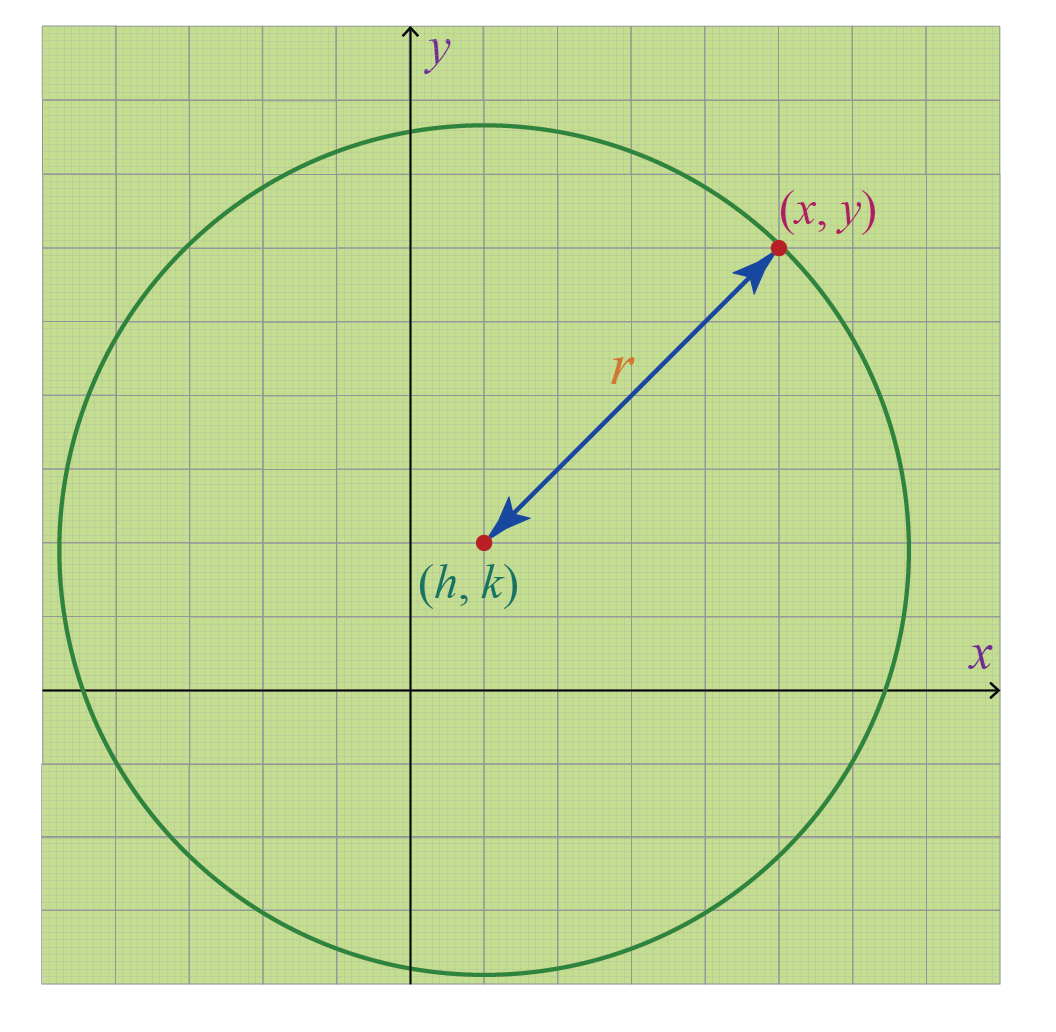The radius of a circle equation in the cartesian coordinate plane is given by:

 $$(x−h)^2 + (y−k)^2 = r^2$$

$$(x,y)$$ are the points on the circumference of the circle which are at a distance ‘$$r$$’ (radius) from the center $$(h,k)$$.

When the center of the circle is at origin $$(0,0)$$, the equation of the circle reduces to:

$x^2 + y^2 = r^2$

Arc of the circle is the distance between two points along a section of a curve. The radius of the curve is the radius of the circle of which it is a part.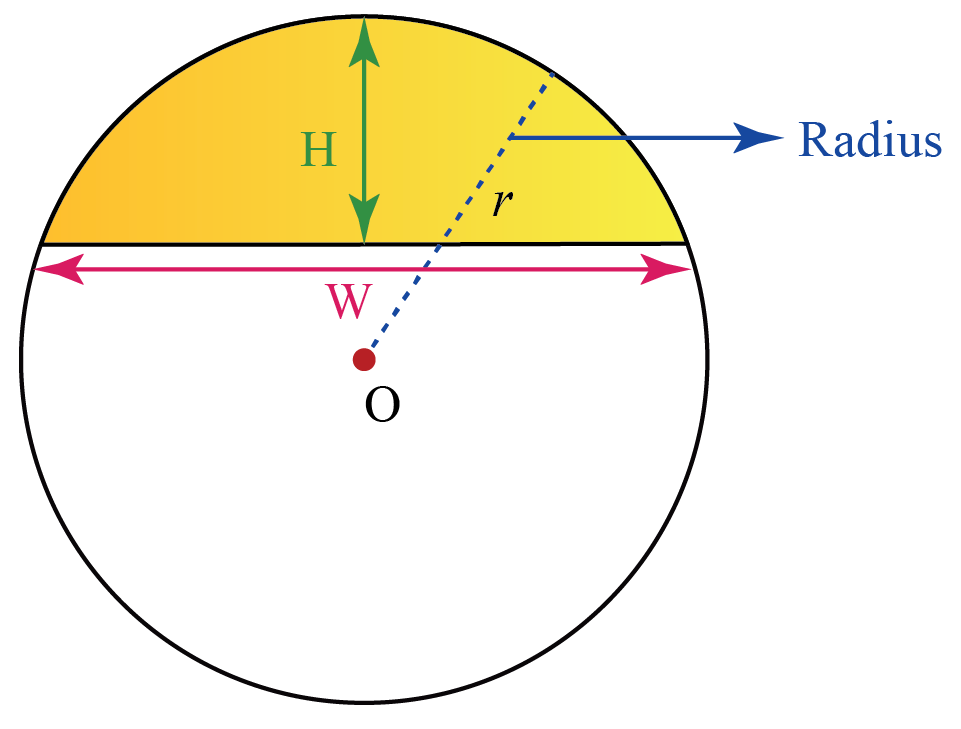The formula to find the radius when the length of the chord defining the base (W) and the height (H) measured at the midpoint of the arc's base is given is:

 $\text{Radius}= \frac{\text{H}}{2} +\frac{\text{W}^2}{8\text{H}}$

Help your child score higher with Cuemath’s proprietary FREE Diagnostic Test. Get access to detailed reports, customized learning plans, and a FREE counseling session. Attempt the test now.

## Solved Examples

 Example 1

The radius of the given circle is $$5 \text{ cm}$$. Find its circumference and area. (Use $$\pi \!= \!3.14$$)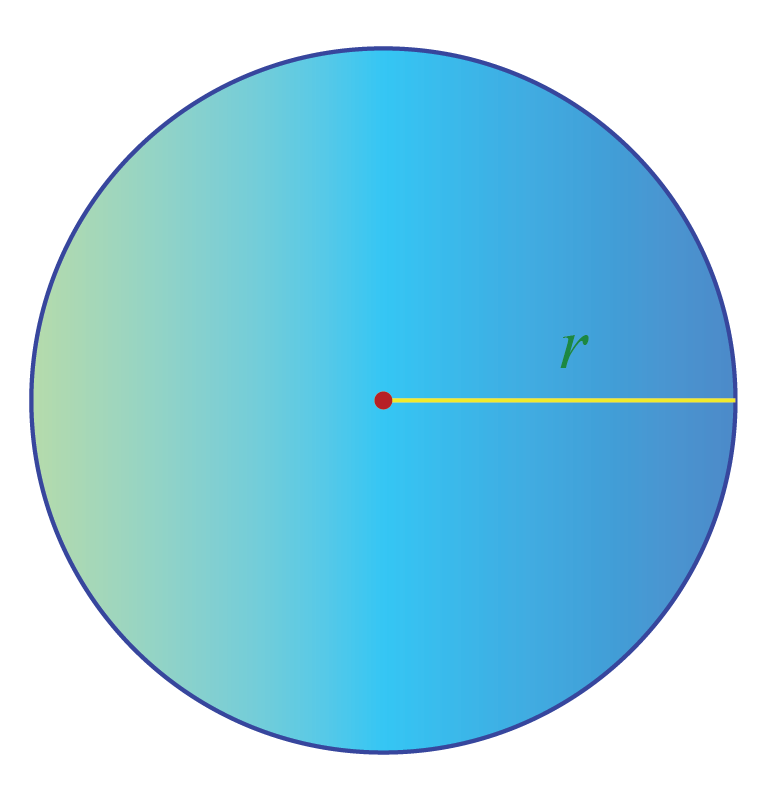Solution:

We know that radius of the given circle $$=5\ \text {cm}$$

We know that circumference

\begin{align} &= 2\pi r \\ &= 2\times \pi \times 5 \\ &=2\times 3.14 \times 5 \\ &=31.4\:\text{cm} \end{align}

We know that area of the circle

\begin{align} &= \pi r^2\\ &=(\pi \times 5 \times 5)\\ &=25 \times 3.14 \\ &=78.5\: \text{cm}^2 \end{align}

 $$\therefore$$  Circumference $$=31.4\:\text{cm}$$  and Area of the circle $$=78.5\: \text{cm}^2$$
 Example 2

The area of a circle is $$12.56\: \text{cm}^2$$. What is its diameter? (Use $$\pi = 3.14$$)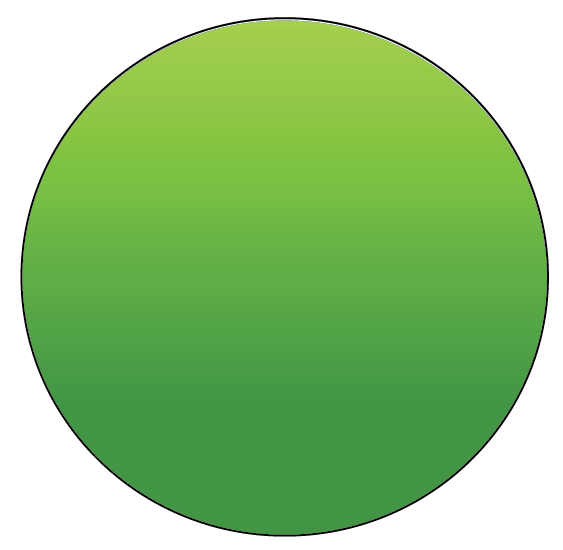Solution:

We know that the area of the circle

\begin{align} &= \pi r^2\\ \therefore 12.56&=(\pi \times r^2)\\ 12.56&=\text{r}^2\times 3.14 \\ r^2&=\frac{12.56}{3.14} \\ r&=\sqrt{\frac{12.56}{3.14}}\\ r&=\sqrt{4}\\ r&=2 \:\text{cm} \end{align}

We know that diameter of a circle

\begin{align}&= 2r\\ &= 2 \times 2 \\ &=4 \:\text{cm} \end{align}

 $$\therefore$$ Diameter of the circle $$= 4 \text{ cm}$$
 Example 3

Amy broke a circular disc exactly into two halves. The radius of the disc is $$14 \:\text{cm}$$. What is the perimeter of the broken half-disc? Use $$\left(\pi = \frac{22}{7}\right)$$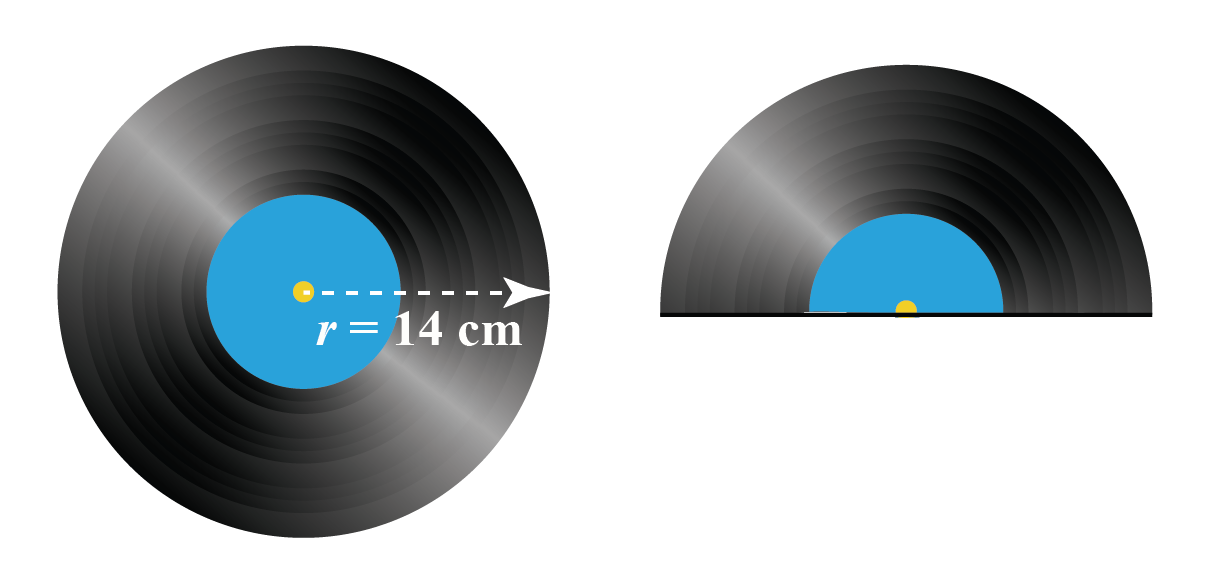Solution:

We know that radius of the given disc

$=14\ \text {cm}$

We know that circumference

\begin{align} &= 2\pi r \\ &= 2\times \pi \times 14 \\ &=2\times \frac{22}{7}\times 14 \\ &=88\:\text{cm} \end{align}

$$88\: \text{cm}$$ is the circumference of the full disc .

The perimeter of the broken disc which is semi-circular in shape is the sum of half the perimeter of the circle and the diameter $(\pi r\! +\!\text{AB})$.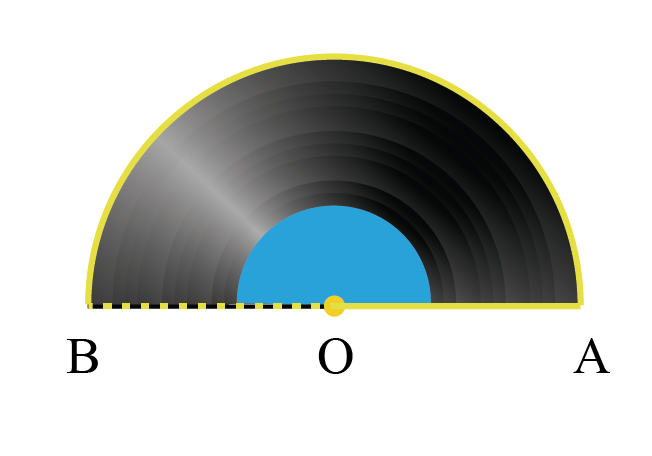\begin{align} &= \frac{88}{2} +14+14\\ &= 28+44 \\ &=72 \\ &=58\:\text{cm} \end{align}

 $$\therefore$$ Perimeter of broken half-disc $$=72\:\text{cm}$$
 Example 4

Find the radius of the circle whose center is O$$(2,1)$$, and the point P$$(5,5)$$ lies on the circumference.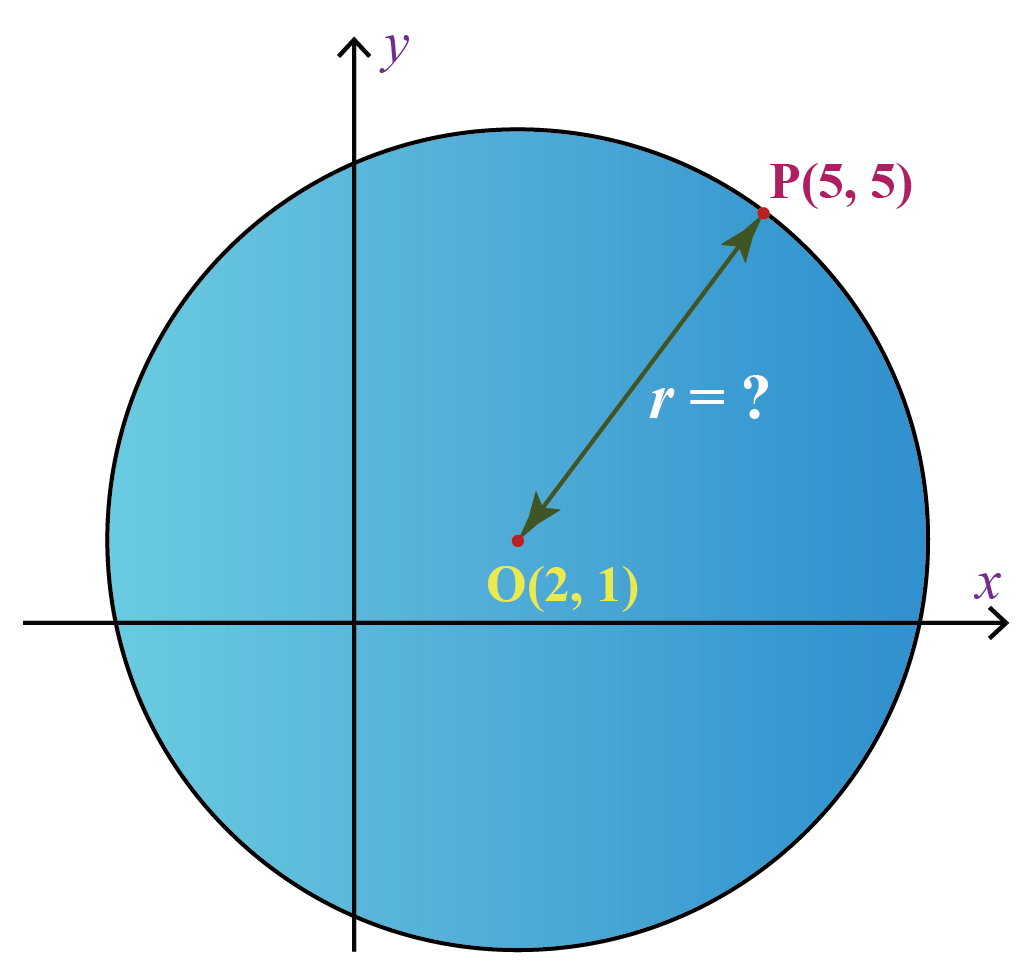Solution:

The equation of a circle in the cartesian plane is given by:

$(x−h)^2 + (y−k)^2 = r^2$

Substituting the value of $$(x,y)$$  as $$(5, 5)$$ and $$(h,k)$$ as $$(2,1)$$ we get

\begin{align} &= (5−2)^2 + (5-1)^2 = r^2\\ &= (3)^2 + (4)^2= r^2\\ &= 9+16 =r^2\\ r^2&= 25\\ \therefore r&=5 \end{align}

 $$\therefore$$ Radius of the given circle is $$5 \:\text{units}$$
 Example 5

The dimensions of the segment of a circle are as shown in the figure. Find the radius of the curve.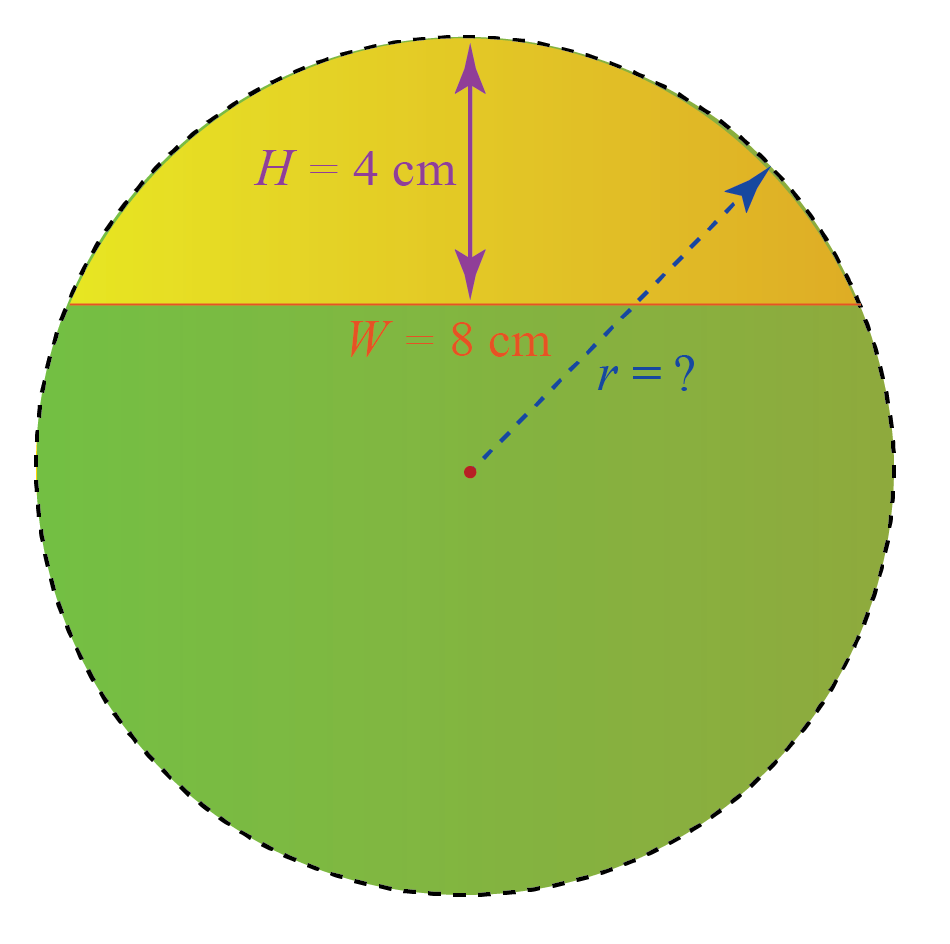Solution:

$= \frac{\text{H}}{2} +\frac{\text{W}^2}{8\text{H}}$

Given

\begin{align} \text{W} &= 8\\ \text{H}&=4 \end{align}

Substituting the above values in the radius of a curve formula, we get:

\begin{align} &= \frac{ {4}}{2} +\frac{8^2}{8\times\text{4}} \\ &=2 +\frac{64}{32}\\ &=2+2\\ &=4 \end{align}

 $$\therefore$$ Radius of the curve is $$4\: \text{cm}$$

## Practice QuestionsChallenging Questions
1.  Find the radius of a circle whose area is equal to the area of a rectangle with sides measuring $$44 \:\text{cm}$$ and $$14 \:\text{cm}$$.
2. The ratio of radii of two circles is $$2:3$$. Find the ratio of their circumference.
3. John cut out a circle of radius $$5 \:\text{cm}$$ from a square sheet of side $$10 \:\text{cm}$$. What is the area of the remaining sheet?

IMO (International Maths Olympiad) is a competitive exam in Mathematics conducted annually for school students. It encourages children to develop their math solving skills from a competition perspective.

## 1. How do you find the radius of a circle?

The radius of a circle can be found when the diameter, circumference, or the area are known.

• \begin{align}\text{Radius}= \frac{\text{Diameter}}{2} \end{align}
• \begin{align}\text{Radius}= \frac{\text{Circumference }}{2\pi} \end{align}
• \begin{align}\text{Radius}= \sqrt \frac{\text{Area of the circle}}{\pi} \end{align}

## 2. How to calculate the radius of a circle?

If the diameter of the circle is known, we can calculate the radius using the formula: $\text{Radius}= \frac{\text{Diameter}}{2}$

If the circumference is known, we can apply the formula: $\text{Radius}= \frac{\text{Circumference }}{2\pi}$ and calculate the radius.

If the area of the circle is known, we can apply the formula: $\text{Radius}= \sqrt \frac{\text{Area of the circle}}{\pi}$ and find the radius.

## 3. What is radius of a curve?

The radius of a curve is the radius of the circle of which it is a part of.

The formula to find the radius when the length of the chord defining the base (W) and the height measured at the midpoint of the arc's base (H) is:

$\text{Radius}= \frac{\text{H}}{2} +\frac{\text{W}^2}{8\text{H}}$

More Important Topics
Numbers
Algebra
Geometry
Measurement
Money
Data
Trigonometry
Calculus